Author: Oscar Cronquist Article last updated on March 22, 2021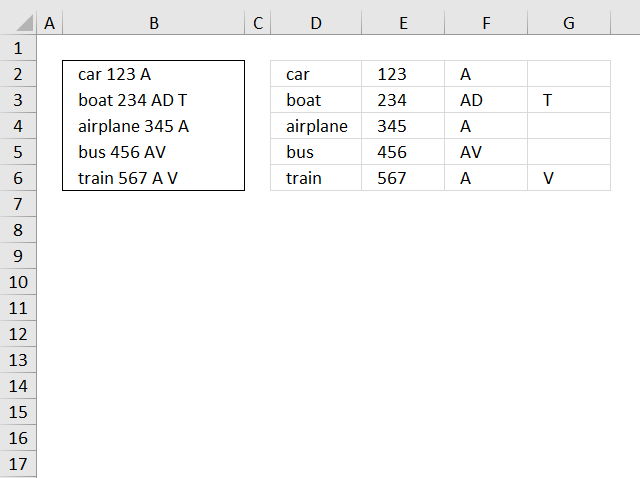This blog article describes how to split strings in a cell with space as a delimiting character, like Text to columns. Text to columns separates the contents of one cell into separate columns.

I present here four different formulas that does the job, I recommend example 3 or example 4. Short and easy to understand.

## 1. Blank as delimiting character - array formulaArray formula in cell D1:

=MID(" "&\$B2&" ", SMALL(IF(ISERROR(SEARCH(MID(" "&\$B2&" ", ROW(\$1:\$99), 1), " ")), "", ROW(\$1:\$99)+1), COLUMN(A1)), SMALL(IF(ISERROR(SEARCH(MID(" "&\$B2&" ", ROW(\$1:\$99), 1), " ")), "", ROW(\$1:\$99)), COLUMN(A1)+1)-SMALL(IF(ISERROR(SEARCH(MID(" "&\$B2&" ", ROW(\$1:\$99), 1), " ")), "", ROW(\$1:\$99)), COLUMN(A1))-1)

To enter an array formula, type the formula in a cell then press and hold CTRL + SHIFT simultaneously, now press Enter once. Release all keys.

The formula bar now shows the formula with a beginning and ending curly bracket telling you that you entered the formula successfully. Don't enter the curly brackets yourself.

Copy cell D2 and paste to cell range D2:G6.

### Explaining formula in cell D1

#### Step 1 - Split characters in a cell into an array

The MID function returns a substring from a string based on the starting position and the number of characters you want to extract. The ROW function returns an array from 1 to 99.

MID(" "&\$B2&" ", ROW(\$1:\$99), 1)

becomes

MID(" car 123 A ", {1; 2; 3; 4; 5; 6; 7; 8; 9; 10; 11; ... ; 99}, 1)

and returns this array:

{" "; "c"; "a"; "r"; " "; "1"; "2"; "3"; " "; "A"; ... ; ""}

#### Step 2 - Search for a blank as a delimiting character and return position

The SEARCH function returns the number of the character at which a specific character or text string is found reading left to right (not case-sensitive), if string is not found the function returns an error value. The ISERROR function returns TRUE if the value is an error.

The IF function has three arguments, the first one must be a logical expression. If the expression evaluates to TRUE then one thing happens (argument 2) and if FALSE another thing happens (argument 3). If the ISERROR function returns FALSE the function returns the corresponding row number.

The SMALL function then returns the k-th smallest value in the array based on the column function and a relative cell reference that changes when the cell is copied to the next cell. This makes the formula return a new value in each cell.

SMALL(IF(ISERROR(SEARCH(MID(" "&\$B2&" ", ROW(\$1:\$99), 1), " ")), "", ROW(\$1:\$99)), COLUMN(A1))

becomes

SMALL(IF(ISERROR(SEARCH({" "; "c"; "a"; "r"; " "; "1"; "2"; "3"; " "; "A"; ... ; ""}, " ")), "", ROW(\$1:\$99)), COLUMN(A1))

I have shortened the arrays to save space.

becomes

SMALL(IF(ISERROR({1; #VALUE!; #VALUE!; #VALUE!; 1; #VALUE!; #VALUE!; #VALUE!; 1; #VALUE!; ... ; 1}), "", ROW(\$1:\$99)), COLUMN(A1))

becomes

SMALL(IF(ISERROR({1; #VALUE!; #VALUE!; #VALUE!; 1; #VALUE!; #VALUE!; #VALUE!; 1; #VALUE!; ... ; 1}), "", ROW(\$1:\$99)), COLUMN(A1))

becomes

SMALL({1; ""; ""; ""; 5; ""; ""; ""; 9; ""; ... ; 99}, COLUMN(A1))

becomes

SMALL({1; ""; ""; ""; 5; ""; ""; ""; 9; ""; ... ; 99}, 1)

and returns 1.

#### Step 3 - Calculate length argument in mid function

SMALL(IF(ISERROR(SEARCH(MID(" "&\$B2&" ", ROW(\$1:\$99), 1), " ")), "", ROW(\$1:\$99)), COLUMN(A1)+1)-SMALL(IF(ISERROR(SEARCH(MID(" "&\$B2&" ", ROW(\$1:\$99), 1), " ")), "", ROW(\$1:\$99)), COLUMN(A1)))

SMALL({1; ""; ""; ""; 5; ""; ""; ""; 9; ""; ... ; 99}, 2)-SMALL({1; ""; ""; ""; 5; ""; ""; ""; 9; ""; ... ; 99}, 1)

becomes

5-1 equals 4.

#### Step 4 - Filter word

=MID(" "&\$B2&" ", SMALL(IF(ISERROR(SEARCH(MID(" "&\$B2&" ", ROW(\$1:\$99), 1), " ")), "", ROW(\$1:\$99)), COLUMN(A1)), SMALL(IF(ISERROR(SEARCH(MID(" "&\$B2&" ", ROW(\$1:\$99), 1), " ")), "", ROW(\$1:\$99)), COLUMN(A1)+1)-SMALL(IF(ISERROR(SEARCH(MID(" "&\$B2&" ", ROW(\$1:\$99), 1), " ")), "", ROW(\$1:\$99)), COLUMN(A1)))

becomes

=MID(" "&\$B2&" ", 1, 4)

becomes

MID(" car 123 A ", 1, 4)

and returns "car" in cell D2.

## 2 Any delimiting character - array formula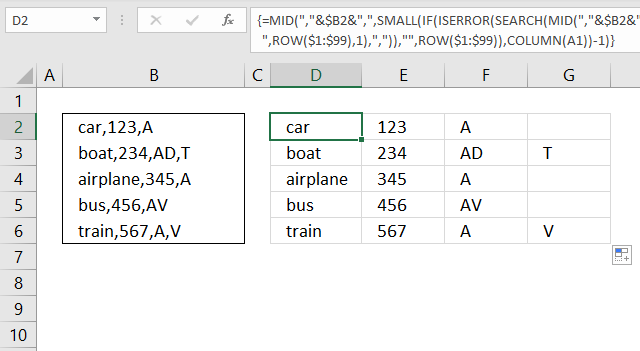The formula in cell D2 in the image above shows how to split a text string using a comma but you can use any number of characters if you like.

For example, replace this "," with the delimiting characters you want to use. Perhaps you want to use three characters example: " | ",  make sure you add double quotes before and after. Replace all instances of "," with " | " in the formula below.

=MID(","&\$B2&",", SMALL(IF(ISERROR(SEARCH(MID(","&\$B2&",", ROW(\$1:\$99),1), ",")),"", ROW(\$1:\$99)+1), COLUMN(A1)), SMALL(IF(ISERROR(SEARCH(MID(","&\$B2&",", ROW(\$1:\$99),1), ",")), "", ROW(\$1:\$99)), COLUMN(A1)+1)-SMALL(IF(ISERROR(SEARCH(MID(","&\$B2&",", ROW(\$1:\$99), 1), ",")), "", ROW(\$1:\$99)), COLUMN(A1))-1)

## 3. Blank as a delimiting character - Excel 365 formula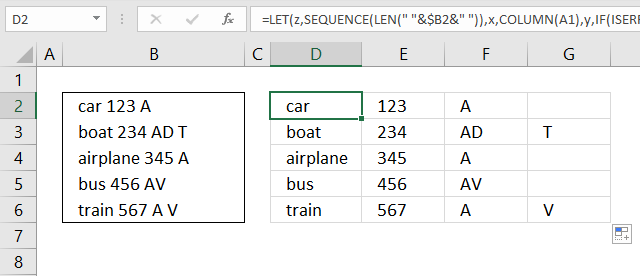The formula in cell D2 is almost identical to the one in example 1, however, the Excel 365 LET function shortens the formula considerably. The only thing that changed is that I am using the SEQUENCE function instead of the ROW function. The SEQUENCE function works with smaller appropriately sized arrays which makes this formula more efficient.

=LET(z, SEQUENCE(LEN(" "&\$B2&" ")), x, COLUMN(A1), y, IF(ISERROR(SEARCH(MID(" "&\$B2&" ", z, 1), " ")), "", z+1), IFERROR(MID(" "&\$B2&" ", SMALL(y, x), SMALL(y, x+1)-SMALL(y, x)-1), ""))

The formula above is entered as a regular formula and it works only in Excel 365.

## 4 Comma as a delimiting character - Excel 365 formula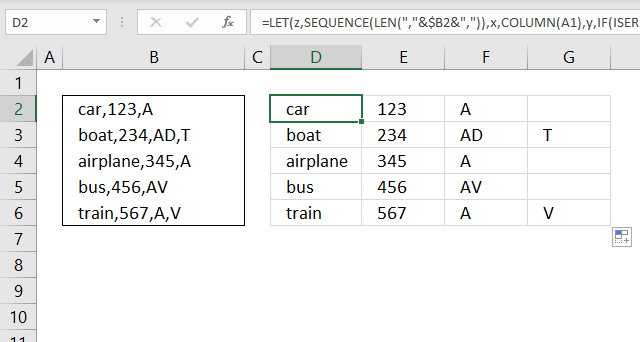The image above demonstrates a formula that splits strings using a comma, however, you can use whatever character or characters you want.

Replace every instance of "," in the formula below with the string you want as a delimiting character. Make sure you use double quotes before and after. For example, " | ".

=LET(z,SEQUENCE(LEN(","&\$B2&",")),x,COLUMN(A1),y,IF(ISERROR(SEARCH(MID(","&\$B2&",",z,1),",")),"",z+1),IFERROR(MID(","&\$B2&",",SMALL(y,x),SMALL(y,x+1)-SMALL(y,x)-1),""))

The formula above is entered as a regular formula and it works only in Excel 365.

## 5. Example 3 - Text to columns with a blank as a delimiting character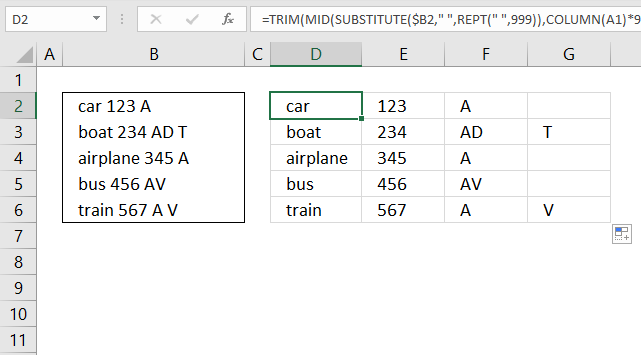This is Rick Rothstein's formula, read his comment.

=TRIM(MID(SUBSTITUTE(\$B2," ",REPT(" ",999)),COLUMN(A1)*999-998,999))

### Explaining formula in cell D2

#### Step 1 - Create a string of blanks

The REPT function repeats a given text string.

REPT(" ",999)

I am not going to show 999 blanks here.

#### Step 2 - Substitute each blank with 999 blanks

The SUBSTITUTE function finds a given string in a value and replaces all instances of it with a specified value.

SUBSTITUTE(\$B2," ",REPT(" ",999))

This inserts blanks as padding between words, the TRIM function will remove the padding in a later step.

#### Step 3 - Create a dynamic value

The COLUMN function calculates the column number based on a cell reference. The cell reference used here is a relative cell reference meaning it changes when you copy the formula to adjacent cells.

COLUMN(A1)*999-998

becomes

1*999-998 equals 1.

In cell E2 the cell reference changes to B1

COLUMN(B1)*999-998

becomes

2*999-998

becomes

1998-998 equals 1000.

#### Step 4 - Get substring

The MID function returns a substring from a string based on the starting position and the number of characters you want to extract.

MID(textstart_numnum_chars)

MID(SUBSTITUTE(\$B2," ",REPT(" ",999)),COLUMN(A1)*999-998,999)

becomes

MID(SUBSTITUTE(\$B2," ",REPT(" ",999)),1,999)

The result is a string containing 999 characters containing the first word and a lot of padding.

#### Step 5 - Remove blanks

The TRIM function deletes all blanks or space characters except single blanks between words in a cell value.

TRIM(MID(SUBSTITUTE(\$B2," ",REPT(" ",999)),COLUMN(A1)*999-998,999))

## 6. Example 4 - Text to columns with any delimiting character or characters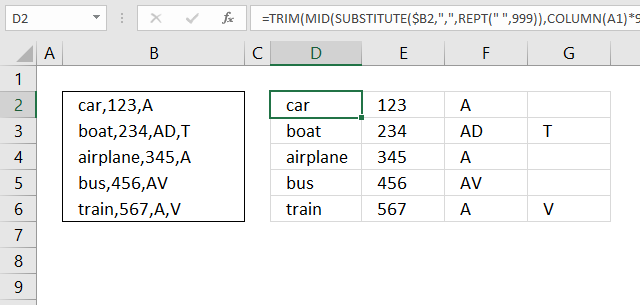This is Rick Rothstein's formula, read his comment. This formula shows how to use a comma as a delimiting character, however, you can use whatever characters you like.

Replace "," with the characters you want to use. For example: " | ", this contains three characters.

=TRIM(MID(SUBSTITUTE(\$B2,",",REPT(" ",999)),COLUMN(A1)*999-998,999))

Se section 5 for an explanation.

## 7. Example 4 - FILTERXML function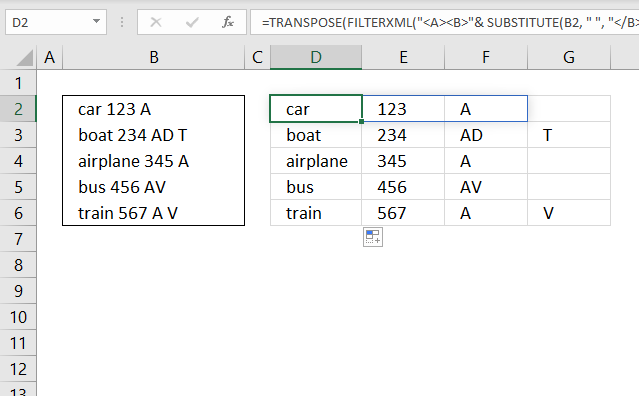The FILTERXML function returns specific data from XML content by using the specified xpath, the function was first introduced in Excel 2013.

=TRANSPOSE(FILTERXML("<A><B>"& SUBSTITUTE(B2, " ", "</B><B>") & "</B></A>","//B"))

The formula works only in Excel 2013 and later versions.

## Explaining formula in cell D2

#### Step 1 - Substitute delimiting character with xml

The SUBSTITUTE function finds a given string in a value and replaces all instances of it with a specified value.

SUBSTITUTE(B2, " ", "</B><B>")

becomes

SUBSTITUTE("car 123 A", " ", "</B><B>")

and returns

"car</B><B>123</B><B>A"

#### Step 2 - Append xml

The ampersand character & concatenates strings in an Excel formula.

"<A><B>"& SUBSTITUTE(B2, " ", "</B><B>") & "</B></A>"

becomes

"<A><B>"& "car</B><B>123</B><B>A" & "</B></A>"

and returns

"<A><B>car</B><B>123</B><B>A</B></A>"

#### Step 3 - Filter xml

The FILTERXML function returns specific data from XML content by using the specified xpath.

FILTERXML("<A><B>"& SUBSTITUTE(B2, " ", "</B><B>") & "</B></A>","//B")

becomes

FILTERXML("<A><B>car</B><B>123</B><B>A</B></A>","//B")

and returns

{"car";123;"A"}

#### Step 4 - Transpose values

The TRANSPOSE function allows you to convert a vertical range to a horizontal range, or vice versa.

TRANSPOSE(FILTERXML("<A><B>"& SUBSTITUTE(B2, " ", "</B><B>") & "</B></A>","//B"))

becomes

TRANSPOSE({"car";123;"A"})

and returns

{"car",123,"A"}